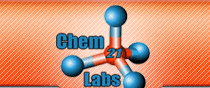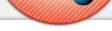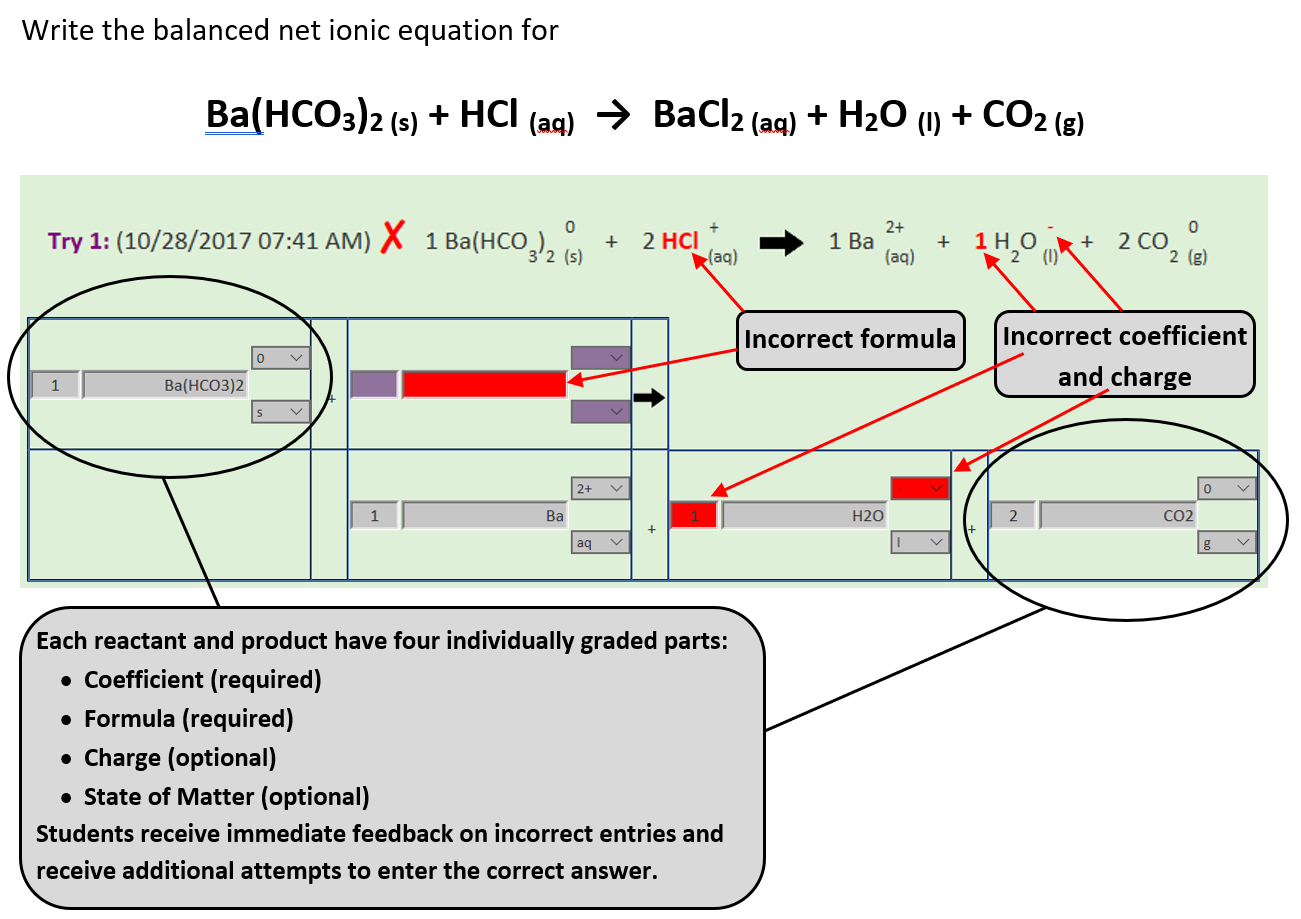Online Lab Submission Students: Instructors: Digital Labs Testimonials Royalties Embedding Content Images, Videos, Webpages, Animations Interactive Spectra Grading Essays / Images Web Reports Display Student Lab Data and Results Automatic Grading of Reported Precision of Lab Data (Decimal Places) Automatic Grading of Reported Significant Figures of Lab Calculations Automatic Grading of Accuracy and Precision of Lab Results Lab Practical / Snow Day / Lab Final Cheating Virtual Labs: Pre-Lab Assignments Interactive Animations: Balancing Chemical Equations The ability to write and balance molecular, ionic and net ionic equations is an essential skill in chemistry. Immediate feedback while practicing this skill is a critical component in the learning process. Each reactant and product in a chemical equation has 4 parts: Coefficient (required) Formula (required) Charge (optional) State of Matter (optional) As the student enters these "parts" for the reactants and products in text boxes and dropdown menus, the program compiles in real-time these "parts" into the actual balanced equation where the formula numbers and state of matter are subscripted and the charges are superscripted (see Try 1 in the image). Additionally, the More Information link below gives the information on how to balance molecular, ionic and net ionic equations. This link expands a section of text so as to conserve space on the lab report webpage - this section of text is collapsed using the Hide Information link (displayed once the More Information link is clicked). Directions For Writing Molecular, Ionic, And Net Ionic Equations: Molecular Equation:  balance the molecular equation by predicting the products and changing the coefficients so that all atoms are balanced.  Equations must be balanced to obey the Law of Conservation of Matter. Ionic Equation:  the ionic equation is obtained by breaking apart into ions any strong acid, strong base or soluble salt that has an (aq) after its formula - the ion's new coefficient will equal the coefficient (from the balanced equation) TIMES the ion's subscript (i.e. 2 Na2SO4 becomes 4 Na+ and 2 SO42-). Do Not Break Apart any reactant or product that has an (s) or (l) or (g) after its formula - list it exactly as it appears in the molecular equation. Do Not Break Apart a weak acid or base that has an (aq) after its formula - by definition a weak acid (or base) is not 100% ionized so it is represented as a molecule and not as ions. Net Ionic Equation:  write the net ionic equation by cancelling out any spectator ions (ions that appear on both sides of the ionic equation) and simplifying the coefficients to the smallest whole number ratio (if necessary). Make sure to include the charge and state for the ions and molecules - all molecules have a charge of zero.